Study Materials

# NCERT Solutions for Class 9th Mathematics

Page 7 of 9

## Chapter 13. Surface Areas and Volumes

### Exercise 13.7

EXERCISE 13.7

Assume π = 22/7, unless stated otherwise.

1. Find the volume of the right circular cone with

(i) radius 6 cm, height 7 cm       (ii) radius 3.5 cm, height 12 cm

Solution: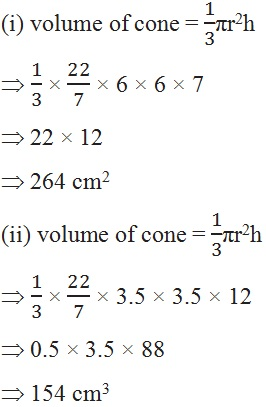2. Find the capacity in litres of a conical vessel with

(i) radius 7 cm, slant height 25 cm   (ii) height 12 cm, slant height 13 cm

Solution:

(i) h2 = 252 - 72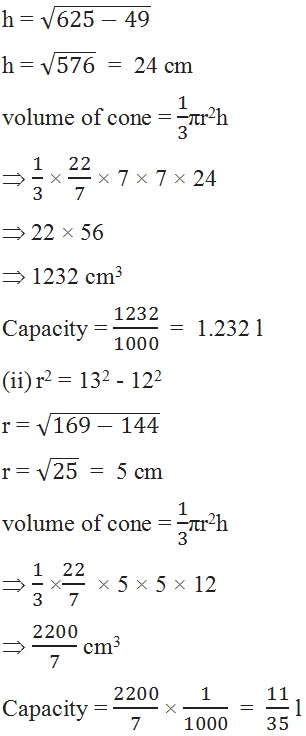3. The height of a cone is 15 cm. If its volume is 1570 cm3, find the radius of the base.(Use π = 3.14)

Solution:

Height = 15 cm,  volume = 1570 cm3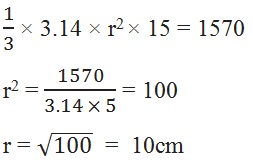4. If the volume of a right circular cone of height 9 cm is 48π cm3, find the diameter of its base.

Solution:

Height = 9 cm,  volume = 48π cm3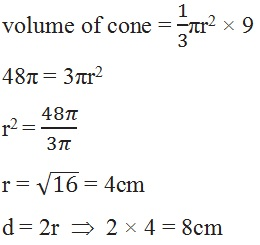5. A conical pit of top diameter 3.5 m is 12 m deep. What is its capacity in kilolitres?

Solution:

Diameter = 3.5 cm ,  height = 12 cm ,  radius = 1.75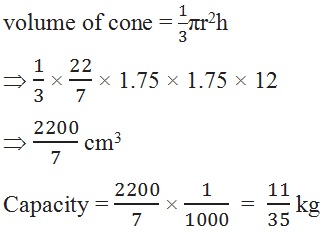6. The volume of a right circular cone is 9856 cm3. If the diameter of the base is 28 cm, find

(i) height of the cone                          (ii) slant height of the cone

(iii) curved surface area of the cone

Solution:

Volume = 9856 cm3 ,  diameter = 28 cm ,  radius = 14cm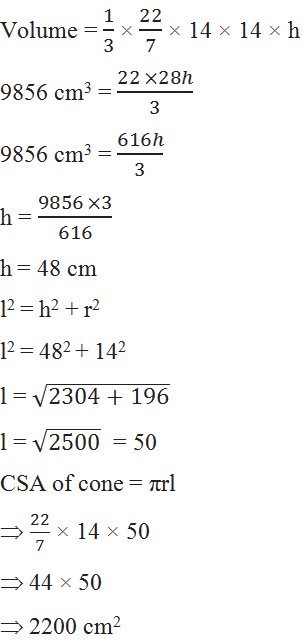7. A right triangle ABC with sides 5 cm, 12 cm and 13 cm is revolved about the side 12 cm. Find the volume of the solid so obtained.

Solution:

Height = 12 cm  ,  radius = 5 cm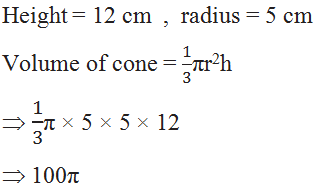8. If the triangle ABC in the Question 7 above is revolved about the side 5 cm, then find the volume of the solid so obtained. Find also the ratio of the volumes of the two solids obtained in Questions 7 and 8.

Solution:

Height = 5 m  ,  radius = 12 m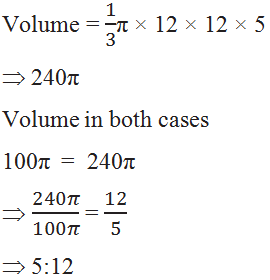9. A heap of wheat is in the form of a cone whose diameter is 10.5 m and height is 3 m. Find its volume. The heap is to be covered by canvas to protect it from rain. Find the area of the canvas required.

Solution:

Diameter = 10.5 m,  height = 3 m ,  radius = 5.25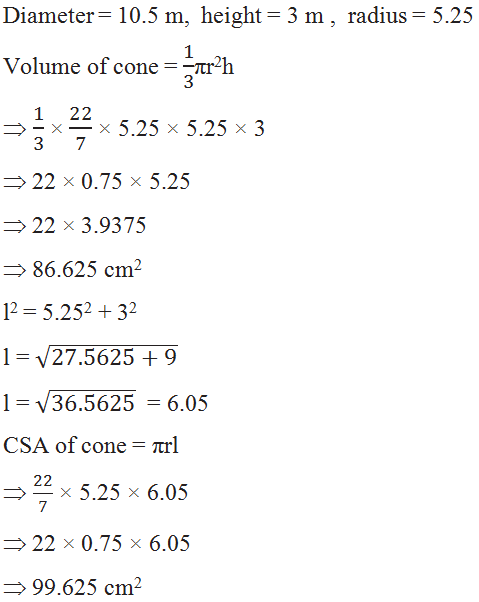Page 7 of 9

Chapter Contents: